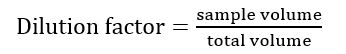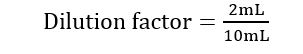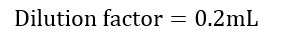Ontario Tech acknowledges the lands and people of the Mississaugas of Scugog Island First Nation.

We are thankful to be welcome on these lands in friendship. The lands we are situated on are covered by the Williams Treaties and are the traditional territory of the Mississaugas, a branch of the greater Anishinaabeg Nation, including Algonquin, Ojibway, Odawa and Pottawatomi. These lands remain home to many Indigenous nations and peoples.

We acknowledge this land out of respect for the Indigenous nations who have cared for Turtle Island, also called North America, from before the arrival of settler peoples until this day. Most importantly, we acknowledge that the history of these lands has been tainted by poor treatment and a lack of friendship with the First Nations who call them home.

This history is something we are all affected by because we are all treaty people in Canada. We all have a shared history to reflect on, and each of us is affected by this history in different ways. Our past defines our present, but if we move forward as friends and allies, then it does not have to define our future.

# Dilutions

## DILUTIONS AND DILUTION FACTORS

For questions pertaining to dilutions, we use the equation:

Dilution = sample volume: total volume

For questions pertaining to dilution factors, we use the equation:Sample volume is the volume of the solution being diluted; total volume is the sample volume in addition to the volume of the diluent.

Example: What is the dilution factor when 2 ml of a sample is mixed in 8mL of water?

Solution: This question asks us to find the dilution factor of a solution, so we use the equation for finding the dilution factor:Our sample volume in the question is 2 mL. We can get the total volume by adding our sample volume (2mL) to the volume of water (8mL). The total volume is found to be 10mL (2mL + 8mL).We have found out dilution factor to be 0.2 X.

Now let's find the dilution of the sample.

Dilution = Sample volume: total volume

Dilution = 2:10 or 1:5

Therefore, we have found the dilution of the sample to be 1:5.

## SERIAL DILUTIONS

For questions pertaining to serial dilutions we use the equation:

DFT= (DF1)*(DF2)*(DF3)...(DF11)

DFT is the total dilution factor of a serial dilution; DFn is the dilution factor of a specific dilution in the series.

Example: What is the dilution factor of a substance to which three 1:5 dilutions have been performed?

Solution: To solve a problem with serial dilutions, we first must find the dilution factor of each dilution using the equation:DF= 1/5 = 0.2

DF= 1/5 = 0.2

DF= 1/5 = 0.2

Now we multiply these to get our total dilution factor for our serial dilution.

DF= 0.2 * 0.2 * 0.2= 0.008

Therefore, the dilution factor for our serial dilutions is 0.008 X.